If you’ve already gone through the “Getting started” vignette, you know about image objects (“cimg” class). This vignette introduces pixsets, which is the other important kind of objects. Pixsets represent sets of pixels, or equivalently binary images, AKA “masks”.

``library(imager)``

# 1 From images to pixsets and back

A pixset is what you get if you run a test on an image:

``````im <- load.example('parrots') %>% grayscale
px <- im > .6 #Select pixels with high luminance
px``````
``Pixel set of size 68993. Width: 768 pix Height: 512 pix Depth: 1 Colour channels: 1 ``
``plot(px)``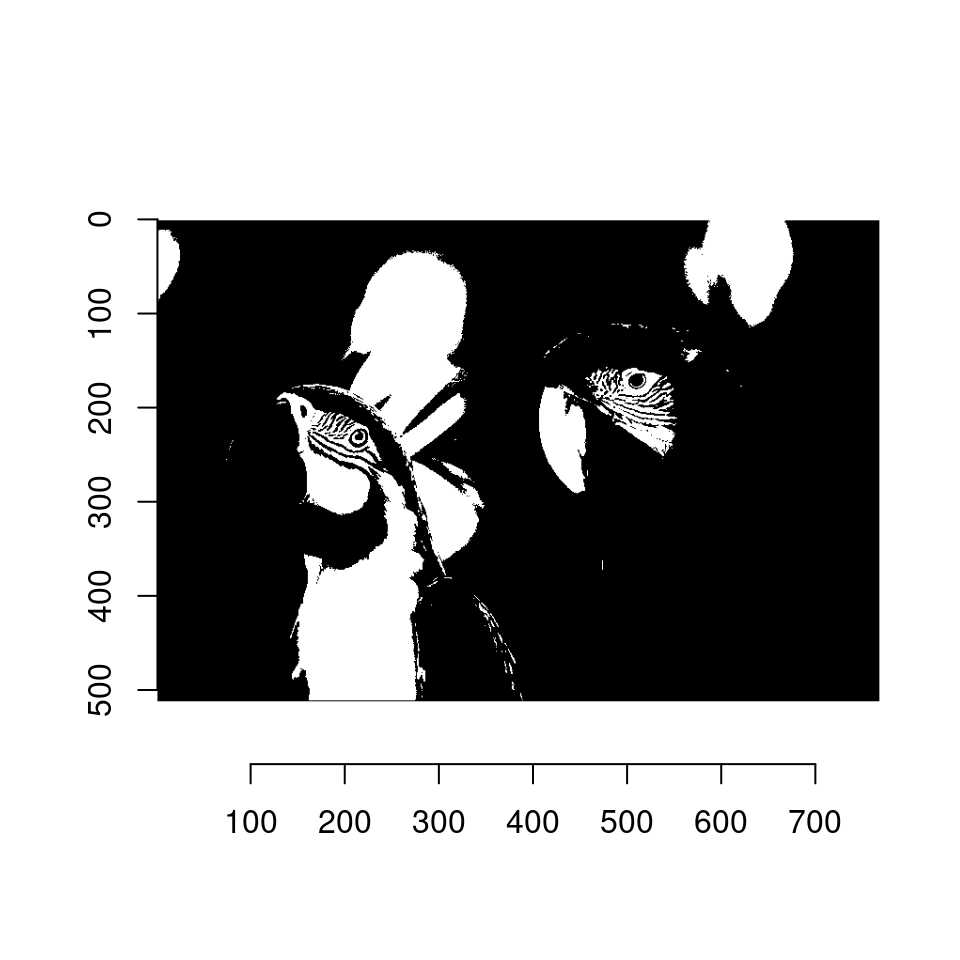Internally, a pixel set is just an array of logical (boolean) values:

``str(px)``
`` 'pixset' logi [1:768, 1:512, 1, 1] FALSE FALSE FALSE FALSE FALSE FALSE ...``

The “TRUE” values correspond to pixels in the set, and “FALSE” to pixels not in the set. The dimensions of the pixset are the same as that of the original image:

``all(dim(px) == dim(im))``
`` TRUE``

To count the number of pixels in the set, use sum:

``sum(px) #Number of pixels in set``
`` 68993``
``mean(px) #Proportion``
`` 0.1754583``

Converting a pixset to an image results in an image of the same size with zeroes and ones:

``as.cimg(px)``
``Image. Width: 768 pix Height: 512 pix Depth: 1 Colour channels: 1 ``
``````##same thing: automatic conversion to a numeric type
px + 0``````
``Image. Width: 768 pix Height: 512 pix Depth: 1 Colour channels: 1 ``

# 2 Indexing using pixsets

You can use pixsets the same way you’d normally use an array of logicals, e.g. for indexing:

``mean(im[px])``
`` 0.7612596``
``mean(im[!px])``
`` 0.3587848``
``which(px) %>% head``
`` 588 589 590 591 592 593``

# 3 Plotting and visualising pixsets

The “highlight” function is a good way of visualising pixel sets:

``````plot(im)
px <- (isoblur(im,4)  > .5 )
highlight(px)``````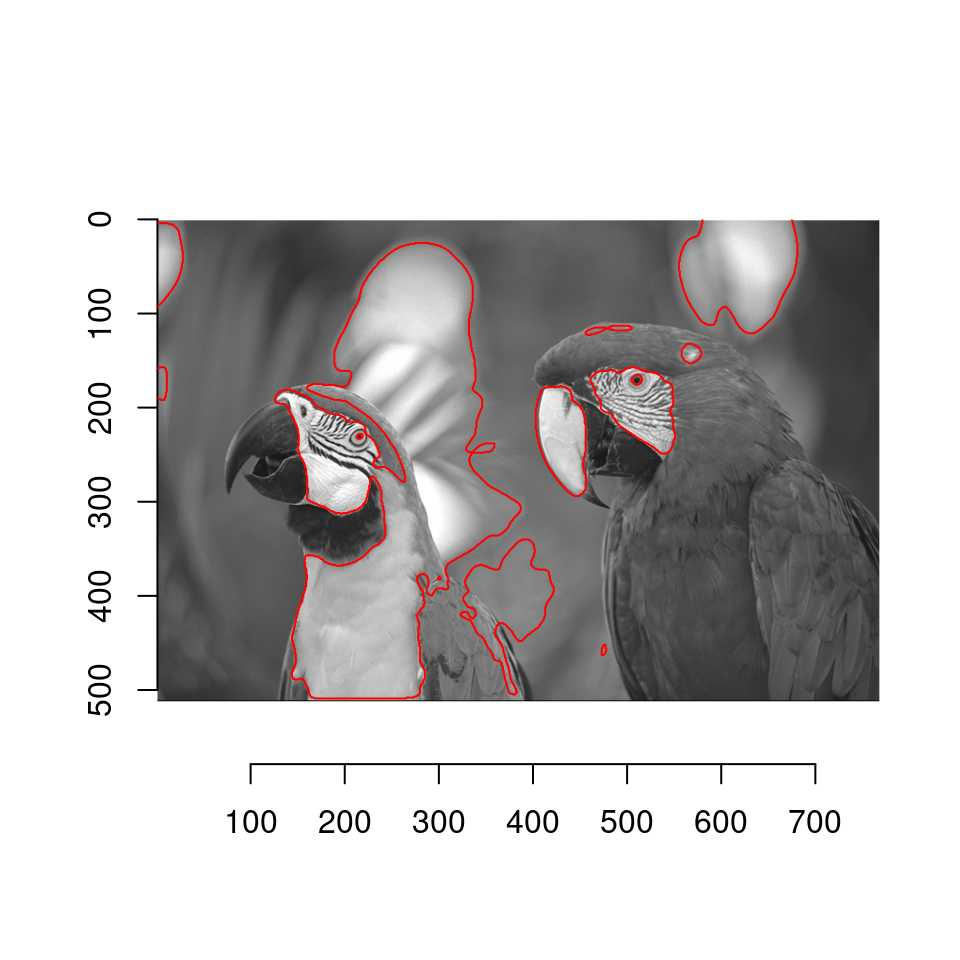highlight extracts the contours of the pixset (see ?contours) and plots them.

colorise is also useful:

``colorise(im,px,"red",alpha=.5) %>% plot``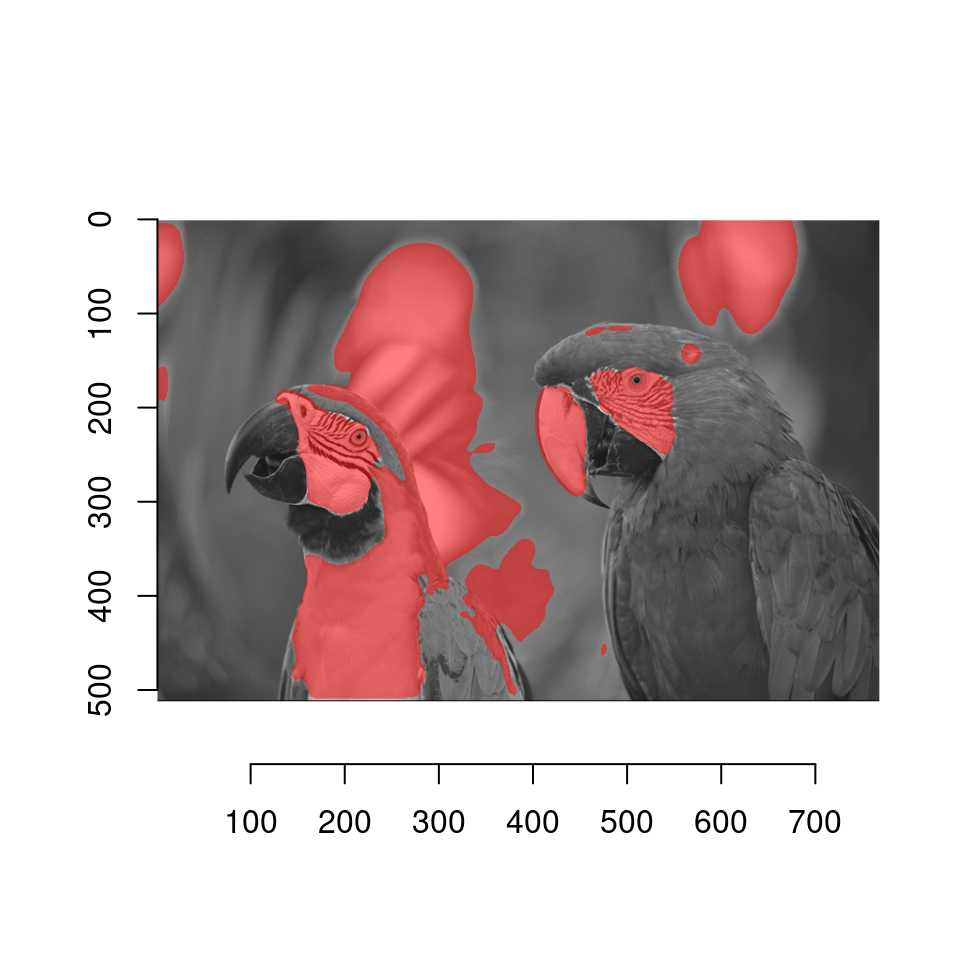You can also use plain old “plot”:

``plot(px)``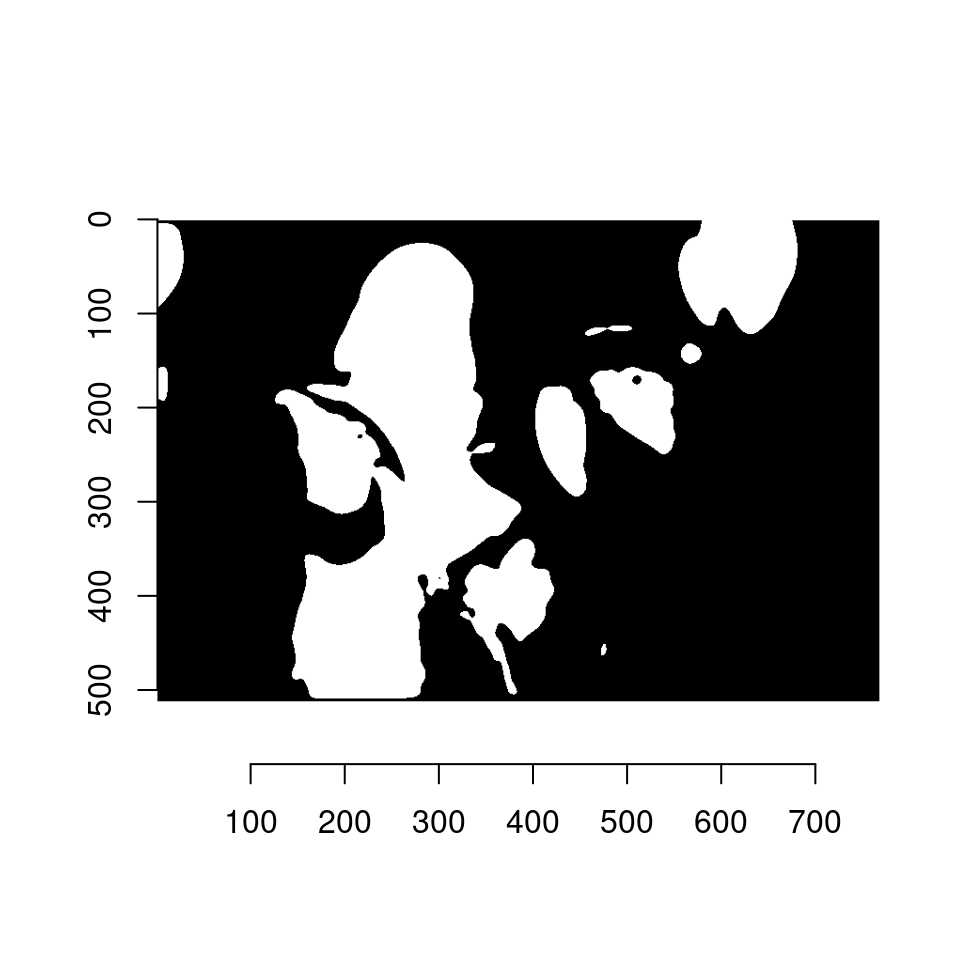It converts “px” to an image and uses plot.cimg.

# 4 Coordinates for pixels in pixsets

The where function returns coordinates for pixels in the set:

``where(px) %>% head``
``````    x y
1 580 1
2 581 1
3 582 1
4 583 1
5 584 1
6 585 1``````

where returns a data.frame. That format is especially convenient if you want to compute some statistics on the coordinates, e.g., the center of mass of a region defined by a pixset:

``where(px) %>% dplyr::summarise(mx=mean(x),my=mean(y))``
``````        mx       my
1 323.3715 244.2327``````

# 5 Selecting contiguous regions, splitting into contiguous regions

In segmentation problems one usually wants contiguous regions: px.flood uses the flood fill algorithm (AKA the bucket tool in image editors) to select pixels based on similarity.

``````plot(im)
#Start the fill at location (180,274). sigma sets the tolerance
px.flood(im,180,274,sigma=.21) %>% highlight``````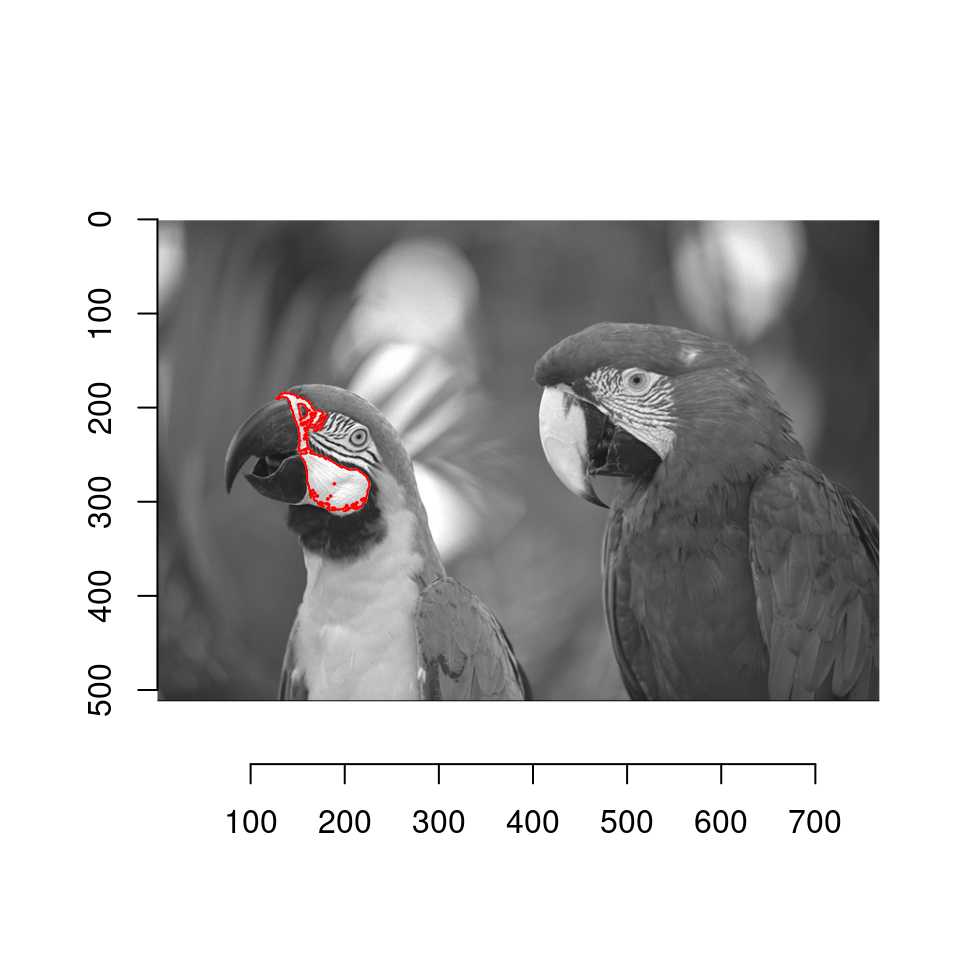It’s also common to want to split a pixset into contiguous regions: use split_connected.

``````sp <- split_connected(px) #returns an imlist
plot(sp[1:4])``````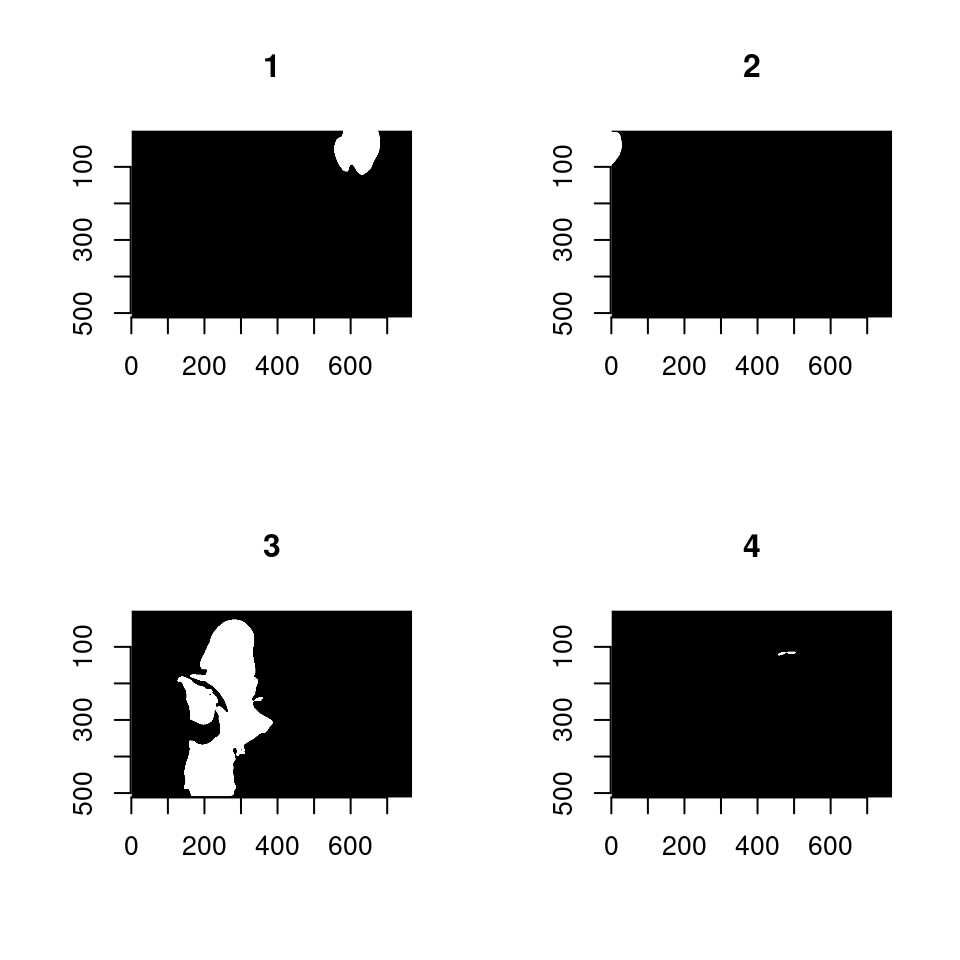``sp``
``Image list of size 10 ``

Each element in the list is a connected pixset. You can use split_connected to check connectedness (there are faster ways, of course, but this is simple):

``````is.connected <- function(px) length(split_connected(px)) == 1
sapply(sp,is.connected)``````
``  TRUE TRUE TRUE TRUE TRUE TRUE TRUE TRUE TRUE TRUE``
``is.connected(px)``
`` FALSE``

Use the “high_connectivity” argument to extend to diagonal neighbours as well. See ?label for more.

# 6 Boundaries

The boundary function computes the boundaries of the set:

``boundary(px) %>% plot``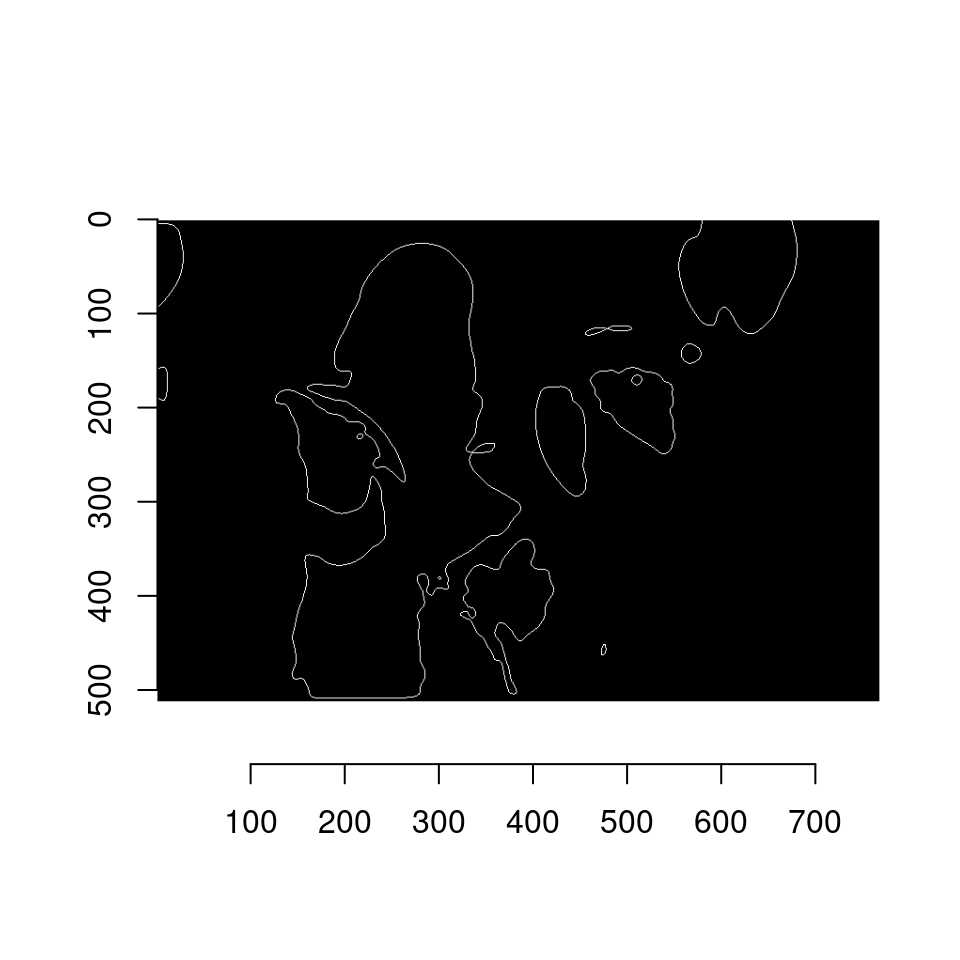``````##Make your own highlight function:
plot(im)
boundary(px) %>% where %\$% { points(x,y,cex=.1,col="red") }``````